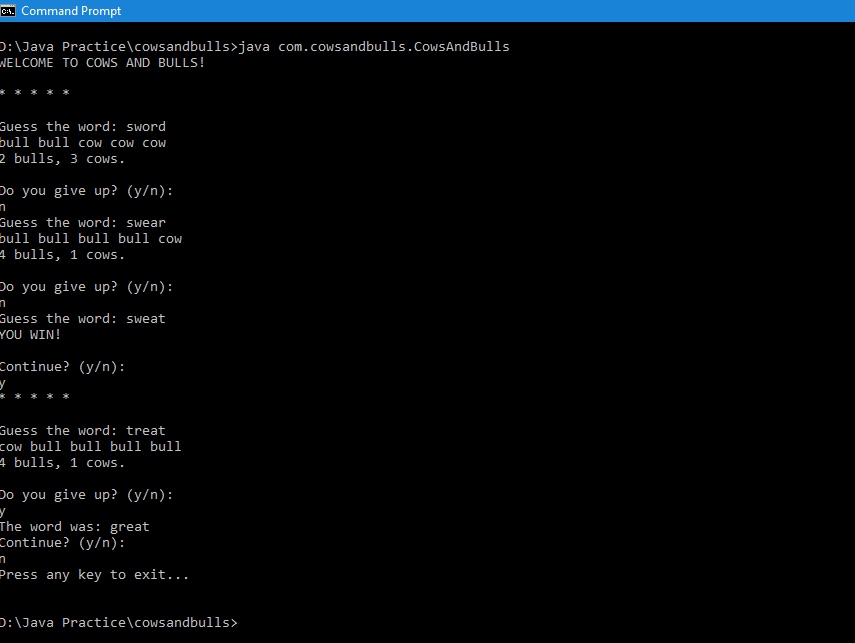# Cows and Bulls: A simple word guessing game

“Cows and Bulls” is a simple word guessing game. In the beginning, the player is told how many letters there are in the word to guess. This is indicated by the number of stars or asterisks displayed. Then the player guesses the word. If the guess is correct, the player is declared a winner and is then prompted to continue or end the game.

If not, the player is told how many hits or “bulls” he made and how many misses or “cows” he made. The player is also asked if he wants to give up.If not, he must continue playing. If he gives up, he will be told what the word was. He will then be prompted to continue or end the game.

One of the subroutines in this program retrieves a random word from a dictionary file which is essentially a text file containing one word per line. It stores all the words in a list and then picks a random element based on the size of the list.

This game has 2 versions: Java and C#

### Java Version

```/*
* To change this template file, choose Tools | Templates
* and open the template in the editor.
*/
package com.cowsandbulls;

import java.io.*;
import java.util.*;
/**
*
* @author Dell
*/
public class CowsAndBulls {

static ArrayList words = new ArrayList();

private static String getRandWord(String path)
{
String randWord = "";

try{

InputStream istream = new FileInputStream("words.txt");

String word;

{
}

int size = words.size();

int idx = (new java.util.Random()).nextInt(size);

randWord = words.get(idx);

}
catch(FileNotFoundException f)
{
System.out.println(f.getMessage());

System.exit(0);
}
catch(IOException io)
{
System.out.println(io.getMessage());

System.exit(0);
}

return randWord;
}

public static void main(String[] args)
{
char cont = '\0';

Scanner sc  = new Scanner(System.in);

System.out.println("WELCOME TO COWS AND BULLS!\n");

do{

String w = getRandWord("words.txt"); //ok
//System.out.println(w);

char[] guessChars = w.toCharArray();

char giveUp = '\0';

for(int i = 0; i&lt; w.length(); i++)
{
System.out.print("* ");
}

System.out.println("\n");

do
{

System.out.print("Guess the word: ");

String guess = sc.nextLine();

if(guess.equals(w))
{
System.out.println("YOU WIN!\n");

break;
}
else
{

if(guess.length() == w.length())
{
int cows = 0, bulls = 0;

for(int i = 0;i &lt; w.length(); i++)
{
if(w.charAt(i) == guess.charAt(i))
{
bulls++;

System.out.print("bull ");
}
else
{
cows++;

System.out.print("cow ");
}
}

System.out.println();

System.out.println(bulls + " bulls, "+cows+" cows.\n");
}
else
{
System.out.println("LENGTH MISMATCH!");
}

System.out.println("Do you give up? (y/n): ");

String s = sc.nextLine();

while(s.length() == 0)
{
System.out.println("Do you give up? (y/n): ");
s = sc.nextLine();
}

giveUp = s.charAt(0);

//giveUp = sc.nextLine().charAt(0);

}
}
while(giveUp != 'y' &amp;&amp; giveUp != 'Y');

if(giveUp == 'y' || giveUp == 'Y')
{
System.out.println("The word was: "+w);
}

System.out.println("Continue? (y/n): ");

String s = sc.nextLine();

while(s.length() == 0)
{
System.out.println("Continue? (y/n): ");
s = sc.nextLine();
}

//

cont = s.charAt(0);

}while(cont == 'y' || cont == 'Y');

System.out.println("Press any key to exit...");

/* */

System.exit(0);

}

}
```

Copy, compile, run and ENJOY!

### C# Version

The code is in the listing.
Also, see the video below.

```using System;
using System.Collections.Generic;
using System.Linq;
using System.Text;

namespace CowsAndBulls
{
class Program
{

private static List lines = new List();

private static string GetWordFromFile(string path)
{

string line;

while ((line = file.ReadLine()) != null)
{
}

int count = lines.Count;

Random r = new Random();

int index = r.Next(count);

string line1 = lines[index];

file.Close();

return line1; //working
}

static void Main(string[] args)
{
//

char cont = '\0';

Console.WriteLine("WELCOME TO COWS AND BULLS!\n");

do
{

string w = GetWordFromFile("..\\..\\words.txt"); //ok

char[] guessChars = w.ToCharArray();

char giveUp = '\0';

//

for (int i = 0; i &lt; w.Length; i++)
{
Console.Write("* ");
}

Console.WriteLine("\n");

do
{

Console.Write("Guess the word: ");

if (guess == w)
{
Console.WriteLine("YOU WIN!\n");

break;
}
else
{

if (guess.Length == w.Length)
{
int cows = 0, bulls = 0;

for (int i = 0; i &lt; w.Length; i++)
{
if (w.Substring(i, 1) == guess.Substring(i, 1))
{
bulls++;

Console.Write("bull ");
}
else
{
cows++;

Console.Write("cow ");
}
}

Console.WriteLine();

Console.WriteLine(bulls + " bulls, " + cows + " cows.\n");
}
else
{
Console.WriteLine("LENGTH MISMATCH!");
}

Console.WriteLine("Do you give up? (y/n): ");

while (s.Length == 0)
{
Console.WriteLine("Do you give up? (y/n): ");
}

giveUp = Convert.ToChar(s.Substring(0, 1));

//

}
}
while (giveUp != 'y' &amp;&amp; giveUp != 'Y');

if (giveUp == 'y' || giveUp == 'Y')
{
Console.WriteLine("The word was: " + w);
}

Console.WriteLine("Continue? (y/n): ");

while (s1.Length == 0)
{
Console.WriteLine("Continue? (y/n): ");
}

//

cont = Convert.ToChar(s1.Substring(0, 1));

} while (cont == 'y' || cont == 'Y');

Console.WriteLine("Press any key to exit...");